# Download Excel Spreadsheets to Calculate Natural Convection Heat Transfer Coefficients

## Newton’s Law of Cooling for Forced and Natural Convection Heat Transfer

Convection heat transfer takes place when a fluid flows past a solid surface, with a difference in temperature between the fluid and the surface. If the fluid flow is due to an external force, like a pump or fan, it is forced convection. If the fluid flow is caused by density differences within the fluid due to internal fluid temperature differences, then it is natural convection, also sometimes called free convection.

An equation that is widely used for both forced and natural convection heat transfer is Newton's Law of Cooling: Q = h A ΔT, where

Q is the rate of heat transfer between the fluid and the surface, Btu/hr (W for S.I.),

A is the area of the surface that is in contact with the fluid, ft2 (m2 for S.I.),

ΔT is the temperature difference between the fluid and the solid surface, oF (oC or K for S.I.),

h is the convective heat transfer coefficient, Btu/hr-ft2oF (W/m2-K for S.I.)

Determining the value for the heat transfer coefficient is the major part of convective heat transfer calculations. The article, "Calculation of Forced Convection Heat Transfer Coefficients with Excel Spreadsheets," provides information about correlations and Excel spreadsheets to calculate heat transfer coefficients for several forced convection heat transfer configurations. This article provides similar information and Excel spreadsheets to calculate natural convection heat transfer coefficients.

## Dimensionless Prandtl, Grashof, Rayleigh and Nusselt Numbers

The equations used to calculate natural convection heat transfer coefficients come from correlations of dimensionless numbers. The dimensionless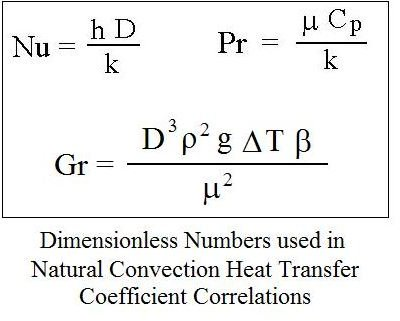numbers typically appearing in these correlations are the Nusselt number, the Prandtl number, the Grashof number, and sometimes the Rayleigh number. The equations for the Nusselt, Prandtl, and Grashof numbers (Nu, Pr, and Gr) are shown in the box at the right. The Rayleigh number is simply: Ra = Gr Pr.

Following are the parameters that appear in these dimensionless numbers (units are given for the U.S engineering system with S.I. units in parentheses):

• D is a characteristic length parameter (e.g. diameter for natural convection from a circular cylinder or a sphere or height of a vertical plate) in ft (m for S.I.)
• ρ is the density of the fluid in slugs/ft3 (Kg/m3 for S.I.)
• μ is the viscosity of the fluid in lb-sec/ft2 (N-s/m2 for S.I.)
• k is the thermal conductivity of the fluid in Btu/hr-ft-oF (W/m-K for S.I.)
• Cp is the heat capacity of the fluid in Btu/lb-oF (J/kg-K for S.I.)
• g is the acceleration due to gravity (32.17 ft/sec2 or 9.81 m/s2)
• β is the coefficient of volume expansion of the fluid in oR (K for S.I.)
• ΔT is the temperature difference between the solid surface and the fluid, oF (oC or K for S.I.)

## Natural Convection Heat Transfer Configurations

The natural convection heat transfer configurations that will be covered in this article are natural convection from:

• a vertical plate
• a horizontal plate
• an inclined plate
• a horizontal cylinder
• a sphere

The following sections will each present the correlation equation(s) for one of these natural convection heat transfer configurations and will present and discuss an Excel spreadsheet to calculate the natural convection heat transfer coefficient using the correlation(s) for that configuration. All of the dimensionless number correlations presented and discussed in this article are described in detail in Reference #1 (Inprocera et al). Several of them are also covered in Reference #2 (Leinhard and Leinhard).

## Natural Convection Heat Transfer from a Vertical Plate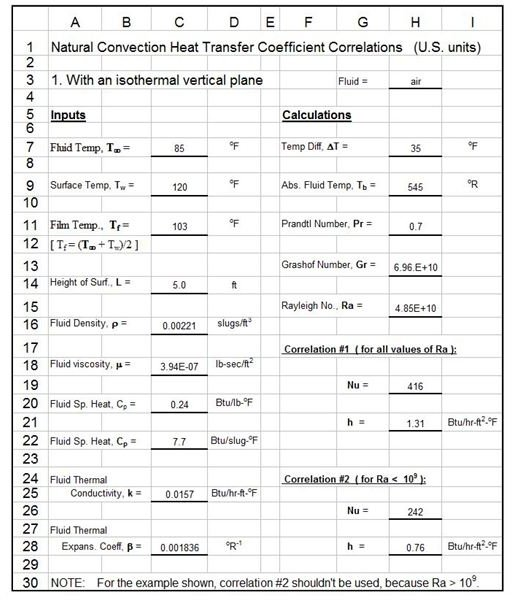An Excel spreadsheet template for calculating heat transfer coefficients for natural convection heat transfer between a fluid and a vertical plate is shown at the left. The dimensionless number correlations for this natural convection heat transfer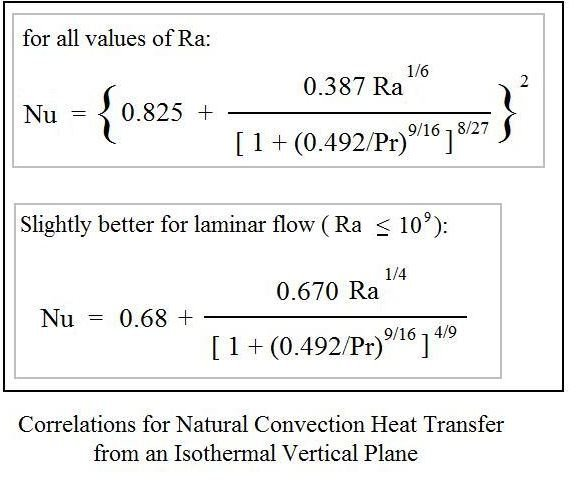configuration are shown in the box at the right. For these correlations, the D in the Nusselt number and Grashof number becomes L, the height of the vertical plate.

The Excel spreadsheet requires input of several parameters such as the height of the vertical plate, the temperatures of the fluid and the plate and values for several fluid properties (density, viscosity, heat capacity, and thermal conductivity). The fluid properties should be evaluated at the film temperature, which is calculated by the spreadsheet as:

film temperature = (fluid temperature + surface temperature)/2

The Excel formulas built into the spreadsheet calculate the absolute film temperature, the thermal expansion coefficient of the fluid, the temperature difference, and the Prandtl, Grashof and Rayleigh numbers. Then it is set to calculate the Nusselt number and the natural convection heat transfer coefficient, h using each of the two correlations shown in the box above.

To download this Excel spreadsheet in either U.S. or S.I. units, click on one of the links below:

## Natural Convection Heat Transfer from a Horizontal Plate

Editor’s Note: It was reported to us by a Plant Engineer that the following spreadsheet contains some errors. We are working on verifying the contents. In the mean time we wanted to inform our readers of the following possible problems:

“1. The Fluid Thermal Expansion Coefficient “beta” is taken as the inverse of the absolute temperature. This is only correct if the fluid is a gas. The correlation is in error for use of water.

2. The Prandtl number calculation is wrong. The units as in the spreadsheet come out to (sec^2-ft)/(ft-slug) and this is supposed to be a dimensionless number. You must change the density input to units of lb/ft^3 and viscosity units to lb-sec/ft^2.

3. The Grashoff number calculation is wrong. The units as in the spreadsheet come out to (ft^2-slugs^2)/(sec^4-lb^2) and this is supposed to be a dimensionless number. You must change the density input to units of lb/ft^3 and viscosity units to lb-sec/ft^2. Then it will work.“

The author of the article has a new website where he may have provided corrections for some of these issues. You may follow him at www.engineeringexcelspreadsheets.com or www.engineeringexceltemplates.com. The original article continues below.

An Excel spreadsheet for natural convection heat transfer between a fluid and a horizontal plate is shown at the right. The dimensionless number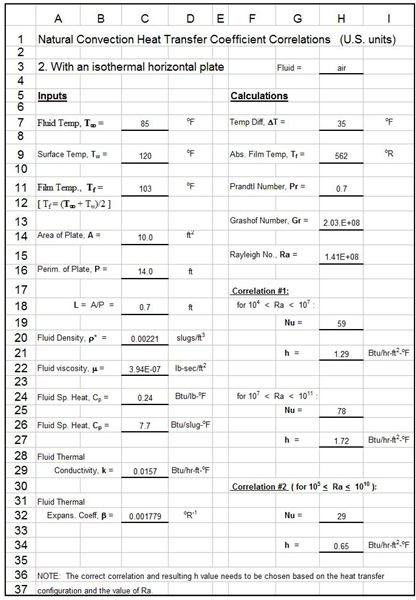correlations for this natural convection configuration, and the applicable range of Rayleigh number for each, are as follows:

For heat transfer from the upper surface of a plate hotter than the fluid or to the lower surface of a plate that is cooler than the fluid:

Nu = 0.54 Ra1/4 ( 104 < Ra < 107 )

Nu = 0.15 Ra1/3 ( 107 < Ra < 1011 )

For heat transfer from the lower surface of a plate hotter than the fluid or to the upper surface of a plate that is cooler than the fluid:

Nu = 0.27 Ra1/4 ( 105 < Ra < 1010 )

The input parameters for this Excel spreadsheet are similar to those needed for natural convection from a vertical plate. One difference is the definition of the length parameter, L, for use in the Nusselt number and the Rayleigh number. For a horizontal plate, the length parameter is defined as: L = area of plate/perimeter of plate, as noted on the spreadsheet. The Excel formulas calculate the Nusselt number and natural convection heat transfer coefficient, h, for each of the three correlations given above. You then need to choose the correct correlation based on the heat transfer configuration and the value of the Rayleigh number.

To download this Excel spreadsheet in either U.S. or S.I. units, click on one of the links below:

## Natural Convection Heat Transfer from an Inclined Plate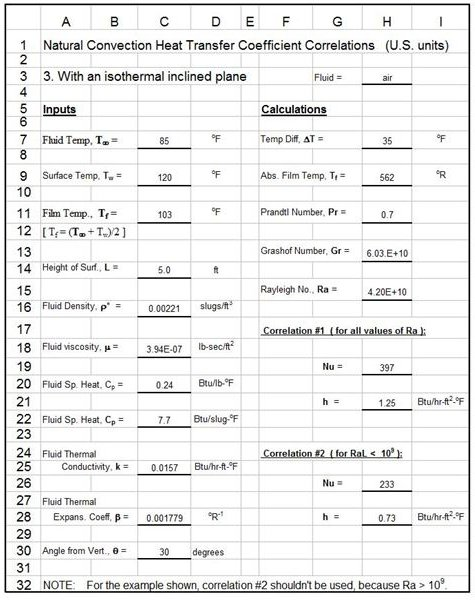Calculations used in the spreadsheet at the left for natural convection heat transfer from an inclined plate are made with the same equations used for a vertical plate, as given and discussed in a previous section on page 2. For an inclined plate, the acceleration due to gravity, g, is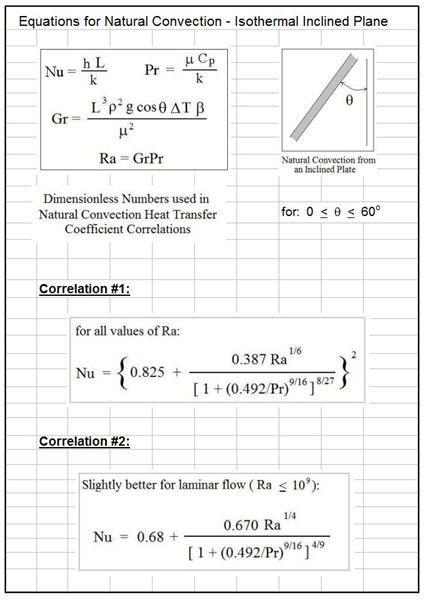replaced by g*cosθ for calculating the Grashof number, Gr, where θ is the angle of the inclined plane from the vertical as shown in the diagram included with the equations for this heat transfer configuration at the right.

The equations shown in the diagram apply to natural convection heat transfer from an inclined plane in the range from 0 < θ < 60o. The input parameters needed are exactly the same as those for vertical plate convection with the addition of the angle of the plate from the vertical, θ. The Excel formulas are set up to calculate Gr using g*cosθ, instead of g. Then the rest of the calculations are the same as for the vertical plate convection configuration.

To download this Excel spreadsheet in either U.S. or S.I. units, click on one of the links below:

## Natural Convection Heat Transfer from a Horizontal Cylinder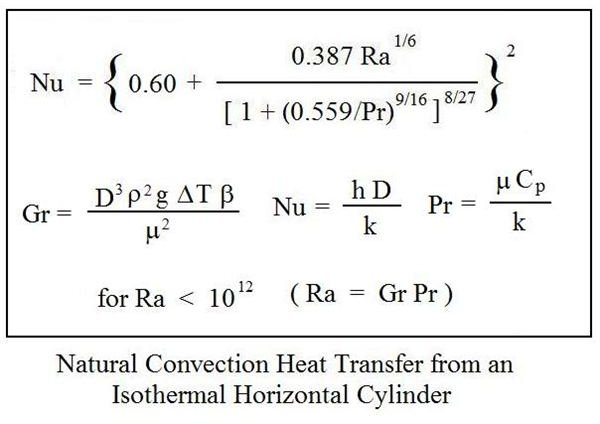The Nusselt number/Rayleigh number/Prandtl number correlation for natural convection heat transfer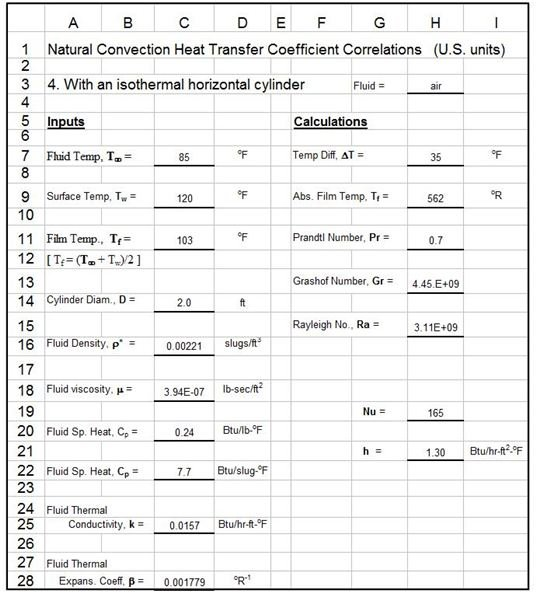between a fluid and an isothermal horizontal cylinder is shown in the box at the left. The Excel spreadsheet image for this configuration is at the right.

As shown in the equations in the box, the length parameter used in the Nusselt number and Grashof number is the cylinder diameter, D. There is a single correlation for the Nusselt number for this configuration. It applies for Rayleigh number less than 1012.

To download this Excel spreadsheet in either U.S. or S.I. units, click on one of the links below:

## Natural Convection Heat Transfer from a Sphere

The Nusselt number/Rayleigh number/Prandtl number correlation for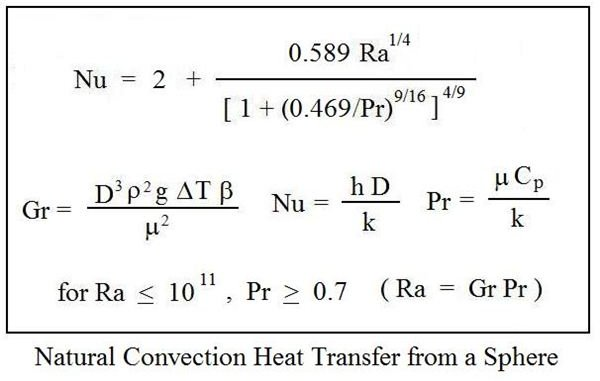natural convection heat transfer between a fluid and an isothermal sphere is shown in the box at the right. The Excel spreadsheet image for natural convection from a sphere is shown at the left.

For natural convection heat transfer from a sphere, the sphere diameter, D, is the length parameter in Nu and Gr. The Nusselt number correlation shown at the right is good for Rayleigh number up to 1011 and Prandtl number of at least 0.7.

To download this Excel spreadsheet in either U.S. or S.I. units, click on one of the links below:

## References

1. Incropera, F.P., DeWitt, D.P, Bergman, T.L., & Lavine, A.S., Fundamentals of Heat and Mass Transfer, 6th Ed., Hoboken, NJ, John Wiley & Sons, (2007).

2. Lienhard, J.H, IV and Lienhard, J.H. V, A Heat Transfer Textbook: A Free Electronic Textbook

3. Bengtson, Harlan H, Fundamentals of Heat Transfer, an online continuing education course for engineering PDH credit

## This post is part of the series: Heat Transfer Fundamentals

Learn about conduction, convection, and radiation, the three mechanisms for heat transfer, including example calculations. Conduction heat transfer is of interest mostly in solids. Convection heat transfer is due to a moving fluid. Heat can also be transferred by electromagnetic radiation.